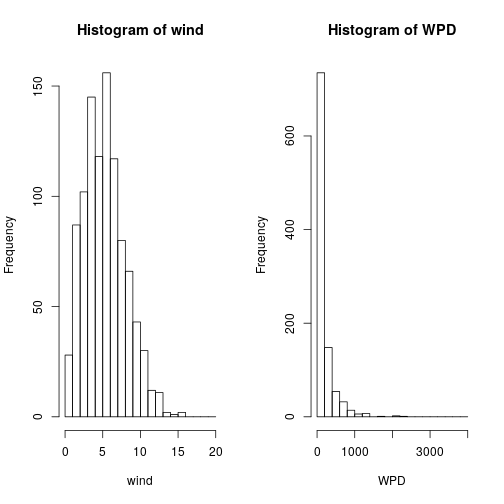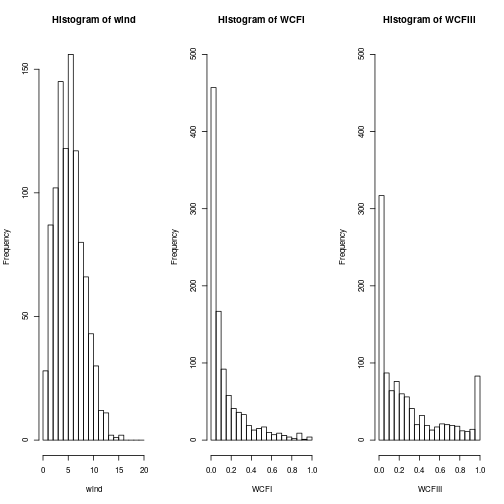Introduction

The energy sector is affected by the atmospheric ciruclation in many ways. On the one hand energy supply from renewable sources like wind, solar or hydropower relies on availability of wind, sunshine or water. On the other hand, energy demand is affected by changes in near-surface temperature. A number of indicators derived from atmospheric variables can be useful as proxies of energy production/demand.

Currently, this package provides two indicators for wind power generation:

1. WindPowerDensity - Wind power that is available for extraction from the wind flow, per square meter of swept area.
2. WindCapacityFactor - Wind power generation of a wind turbine, normalized by the maximum power that the turbine can deliver (rated capacity).

1. Wind Power Density

WindPowerDensity computes the kinetic energy that is available in the wind flow that traverses a unit of area swept by a wind turbine. For an instantaneous wind speed value, it is computed as: WPD = 0.5 * ro * wspd^3 where ro is the air density in Kg/m3 and wspd is the instantaneous wind speed at hub height in m/s. Although wind turbines cannot extract all of the kinetic energy in the wind, and their efficiency can vary substantially at different wind speeds and among different wind turbines, this indicator provides a simple estimation of the wind resource quality. Typically, Wind Power Density is computed over a long period and its mean value is reported.

As an example, we simulate a time series of 1000 wind speed values from a Weibull distribution with scale factor of 6 and a shape factor of 2, which represent a sample of wind speed values obtained at a single location. The Weibull distribution is often assumed to fit observed wind speed values to a probability distribution function. Then, each instantaneous wind speed value is converted to its equivalent WPD. The mean and sd of the WPD can be employed to summarize the wind resource in that location. Otherwise, we can plot the histograms to see the full distribution of values:

library(CSIndicators)
set.seed(1)
wind <- rweibull(n = 1000, shape = 2, scale = 6)
WPD <- WindPowerDensity(wind)
mean(WPD)
##  170.6205
sd(WPD)
##  251.1349
par(mfrow=c(1, 2))
hist(wind, breaks = seq(0, 20))
hist(WPD, breaks = seq(0, 4000, 200))As you can see the histogram of the WPD is highly skewed, even if the wind speed was only a little skewed!

If not specified, an air density of 1.225 kg/m3 is assumed. Otherwise, the parameter ro can be set to a fixed value (for instance the mean air density at the site elevation could be used), or a timeseries of density values measured at each time stamp can be used to obtain more accurate results.

WPD <- WindPowerDensity(wind, ro = 1.15)

2. Wind Capacity Factor

WindCapacityFactor transforms wind speed values into normalized wind power values. The transformation is made employing manufacturer-provided power curves, for five different turbines, as described in Lledó et al. (2019). The generation is normalized by the rated power of the turbine (i.e. the maximum power output it can achieve). This allows for comparisons between turbines of different sizes and wind farms of different installed capacities. Beware that the Capacity Factor (CF) values provided do not take into account any losses due to wakes, electricity transport, blade degradation, curtailments or maintenance shutdowns.

The function allows to choose from five different power curves that are suited for a different range of wind speed conditions. Each of the provided turbines is a representative of a IEC wind class. Generally speaking, commercially available wind turbines can be certified as IEC class I, II, III or a combination of them (I/II and II/III), according to their efficency at different wind speeds and the loads they can withstand. The basic idea is that most turbines in a same IEC class have similar power curves, and the differences of power output can be thoroughly studied with only this set of five turbines.

Notice that power curves are intended to be used with 10-minutal steady wind speed values at hub height, which in modern wind turbines varies between 80 and 120m typically. As the transformation of wind speed into wind power is non-linear, it is recomended to use instantaneous or 10-minutal wind speed values as input. Employing longer period means will produce inaccurate results, as far as the wind is not steady during that period.

Following on the previous example, we will compute now the CF that would be obtained from our sample of 1000 wind speed values when using a turbine of class IEC I, and compare it to the CF values for a class III:

WCFI <- WindCapacityFactor(wind, IEC_class = "I")
WCFIII <- WindCapacityFactor(wind, IEC_class = "III")
par(mfrow=c(1, 3))
hist(wind, breaks = seq(0, 20))
hist(WCFI, breaks = seq(0, 1, 0.05), ylim = c(0, 500))
hist(WCFIII, breaks = seq(0, 1, 0.05), ylim = c(0, 500))par(oldpar)From the CF histograms we can see that, for this particular wind speed distribution, the IEC I turbine (designed for high winds) producess less energy than the IEC III turbine, which is more suitable for this range of wind speed values.

References

• Lledó, Ll., Torralba, V., Soret, A., Ramon, J., & Doblas-Reyes, F.J. (2019). Seasonal forecasts of wind power generation. Renewable Energy, 143, 91–100. https://doi.org/10.1016/j.renene.2019.04.135

• International Standard IEC 61400-1 (third ed.) (2005)